# High School Algebra | MathHelp.com

##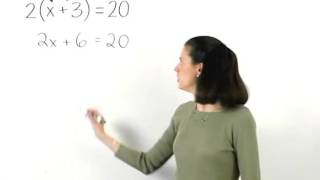By MathHelp.com

This lesson covers perimeter word problems. Students learn to solve "Geometry" word problems involving perimeters of rectangles# Grade 3 Math Perimeter 3 MD 8

##By EngageNY

Solve real world problems involving perimeters of polygons, including finding the perimeter given the side lengths and finding an unknown side length# Area and perimeter problems - rectangular shapes - 3rd/4th grade math

##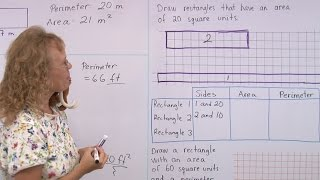By Math Mammoth

After reminding everyone about what is AREA and what is PERIMETER, we solve several problems involving both area and perimeter of rectangles or rectangular shapes.# Linear equation word problems

##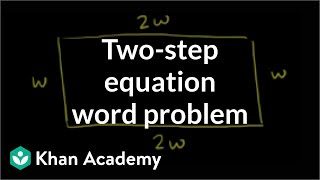By Khan Academy

Here's a nifty word problem in which we find the dimensions of a garden given only the perimeter. Let's create an equation to solve!# Linear equation word problems

##By Khan Academy

Here's a nifty word problem in which we find the dimensions of a garden given only the perimeter. Let's create an equation to solve!# Linear equation word problems

##By Khan Academy

Here's a nifty word problem in which we find the dimensions of a garden given only the perimeter. Let's create an equation to solve!# Part 2 of proof of Heron's formula | Perimeter, area, and volume | Geometry | Khan Academy

##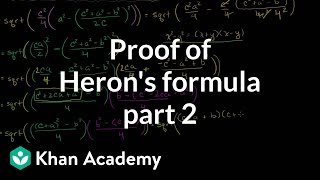By Khan Academy

Video shows that the expression in part 1 is identical to Heron's Formula. This video demonstrates how to use Heron's Formula to determine the area of a triangle while only knowing the lengths of the sides# Part 1 of proof of Heron's formula | Perimeter, area, and volume | Geometry | Khan Academy

##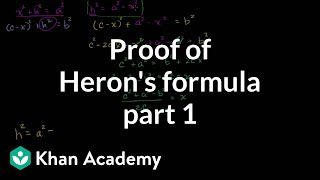By Khan Academy

In this video, the insructor explores and demonsrates the proof of Heron's Formula. The instructor demonstrates with computer software.# Heron's formula | Perimeter, area, and volume | Geometry | Khan Academy

##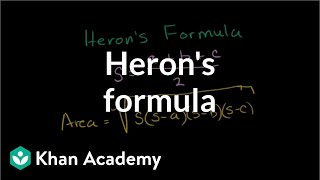By Khan Academy

Using Heron's Formula to determine the area of a triangle while only knowing the lengths of the sides. All Khan Academy content is available for free at www.khanacademy.org# Area of an equilateral triangle | Perimeter, area, and volume | Geometry | Khan Academy

##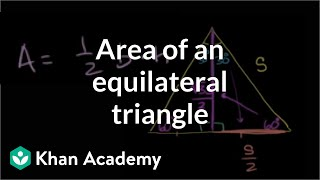By Khan Academy

Finding the formula for the area of an equilateral triangle with side s. All Khan Academy content is available for free at www.khanacademy.org# Volume of a sphere | Perimeter, area, and volume | Geometry | Khan Academy

##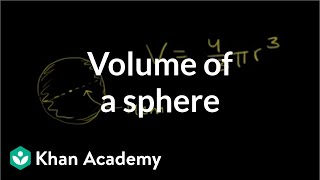By Khan Academy

"Find the volume of a sphere with a diameter of 14cm." Sal Khan solves this problem during this video clip.# Cylinder volume and surface area | Perimeter, area, and volume | Geometry | Khan Academy

##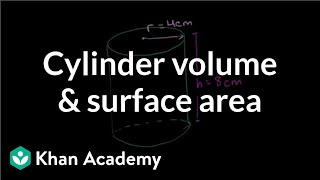By Khan Academy

The other frequently occurring shape is the cylinder. This video will introduce the cylinder and teach you the formulas for finding its surface area and volume.# Labeling parts of a circle | Perimeter, area, and volume | Geometry | Khan Academy

##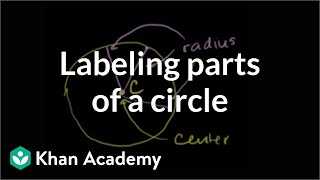By Khan Academy

Learn more: http://www.khanacademy.org/video?v=04N79tItPEA u07_l2_t3_we1 Parts of a Circle Content provided by TheNROCproject.org - (c) Monterey Institute for Technology and Education# Triangle inequality theorem | Perimeter, area, and volume | Geometry | Khan Academy

##By Khan Academy

Salman Khan offers his intuition behind the triangle inequality theorem during this six-minute video lesson.# Basics of Area Perimeter and Volume of Rectangular Shapes.

##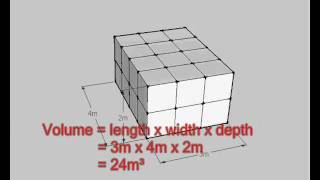By tecmath

This is a basic lesson in working out the perimeter area or volume or rectangular shapes. A house plan is used to show how to find the perimeter of a rectangle. Examples are shown for formulas of perimeter and area.# Rotating 2D shapes in 3D | Perimeter, area, and volume | Geometry | Khan Academy

##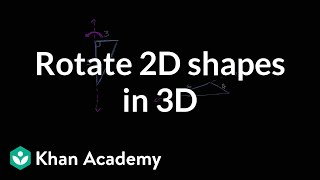By Khan Academy

This video shows the effect of rotating a 2D shape to show the relationship between 2D and 3D shapes.# Interesting perimeter and area problems | Perimeter, area, and volume | Geometry | Khan Academy

##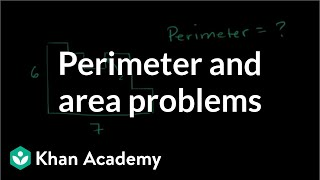By Khan Academy

problems involving perimeter and area# Perimeter and area: the basics | Perimeter, area, and volume | Geometry | Khan Academy

##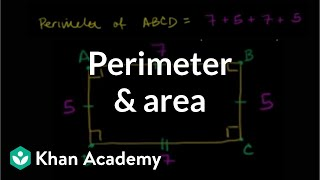By Khan Academy

perimeter and area.# Grade 5 Math Lessons and Worksheets

##By Lumos Learning

It is important for you as a parent to know what your child should be learning in grade 5 math. This is for you to be able to monitor your child’s progress and see if they are having any difficulty with the subject. If you supervise their learning process, then you can determine whether your child needs more help or tutoring. Having a clear idea of the math concepts that your child should be learning also gives you a chance to reinforce their knowledge. Familiarize yourself with the lessons that he or she is learning about so that you can help them anytime they need your assistance. The major topics that are included in grade 5 math are math strands involving whole numbers, decimals, fractions, measurement, plane figures, area and perimeter, ratio, scale, percent, average, graphs, rectangular prisms and cubes, volume, rates and speed, and functions and equations. Your child should develop competency in understanding problems concerning these math concepts. Your child should also be able to translate that knowledge into their problem solving skills. These problems should be applicable in the real world so that your child will be able to visualize and analyze the situation. Grade 5 math already includes using formulas and creating solutions for math problems. This is the introduction of the more complex math concepts and operations. It is preferable that you guide and aid your child as much as you can at this stage. The danger of having your child experience too much difficulty in the subject is that he or she will completely lose interest in math. You should try to avoid this and help your child develop a strong foundation in math concepts. As your child goes on to learn about harder math, you should also be more willing to give them assistance.# Quadrilateral properties | Perimeter, area, and volume | Geometry | Khan Academy

##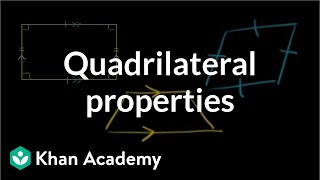By Khan Academy